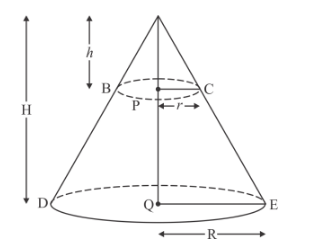# The height of a cone is 60 cm.`
Question:

The height of a cone is $60 \mathrm{~cm}$. A small cone is cut off at the top by a plane parallel to the base and its volume is $\frac{1}{64} t h$ the volume of original cone. The height from the base at which the section is made is:

Solution:

It is given that the height of a cone = 60 cm

The volume of a small cone cut on the top by plane parallel to base of given conevolume of given cone

We have to find the height from the base at which section is made.Let be the radius of base of given cone

Let be the height of given cone

= 60 cm

Let be the radius of base of small cone

Let be the height of small cone

In ΔAPC and ΔAQE

PC || QE

Therefore

$\angle \mathrm{APC}=\angle \mathrm{AQE} \quad[$ Corresponding angles $]$

$\angle \mathrm{ACP}=\angle \mathrm{AEQ} \quad[$ Corresponding angles $]$

Also $\angle \mathrm{PAC}=\angle \mathrm{QAE}$ [Same angle]

Hence ΔAPC ~ ΔAQE

$\frac{A P}{A Q}=\frac{P C}{Q E}$

$\frac{h}{H}=\frac{r}{R}$.......(1)

Volume of cone $\mathrm{ADE}=\frac{1}{3} \pi R^{2} H$

Volume of cone $\mathrm{ABC}=\frac{1}{3} \pi r^{2} h$

$\frac{\text { Volume of cone } \mathrm{ABC}}{\text { Volume of cone } \mathrm{ADE}}=\frac{\frac{1}{3} \pi r^{2} h}{\frac{1}{3} \pi R^{2} H}$

$\frac{1}{64}=\left(\frac{r}{R}\right)^{2} \times \frac{h}{H}$

$\frac{1}{64}=\left(\frac{h}{H}\right)^{2} \times \frac{h}{H}[$ From $(1)]$

$\frac{1}{64}=\left(\frac{h}{H}\right)^{3}$

$\sqrt{\frac{1}{64}}=\frac{h}{H}$

$\frac{1}{4}=\frac{h}{60}$

$h=15$

The height from the base at which section is made $=H-h$

$=60-15 \mathrm{~cm}$

$=45 \mathrm{~cm}$

Hence Option (c) is correct.